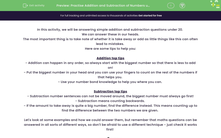# Practise Addition and Subtraction of Numbers up to 20

In this worksheet, students will practise using their addition and subtraction skills and their number bonds to 20.Key stage:  KS 1

Curriculum topic:   Number: Addition and Subtraction

Curriculum subtopic:   Use Addition/Subtraction Facts to 100

Difficulty level:#### Worksheet Overview

In this activity, we will be answering simple addition and subtraction questions under 20.

The most important thing is to take note of whether it is take away or add as little things like this can often lead to mistakes.

- Put the biggest number in your head and you can use your fingers to count on the rest of the numbers if that helps you.

Subtraction top tips

- Subtraction number sentences can not be moved around, the biggest number must always go first!

- Subtraction means counting backwards.

- If the amount to take away is quite a big number, find the difference instead. This means counting up to find the difference between the two numbers we are given.

Let's look at some examples and how we could answer them, but remember that maths questions can be answered in all sorts of different ways, so don't be afraid to use a different technique - just check it works first!Example 1

So, let's put the biggest number first, which is 9, this means we have 6 left to add on.

We need to put 9 in our heads and count on 6 more (hold up 6 fingers if that helps!)

So after 9 it is 10, 11, 12, 13, 14, 15.

We've counted on 6 more numbers to 15, so

6 + 9 = 15

Example 2

Subtract  15 - 9 =

You could use find the difference here as 9 is quite a lot to take away.

To find the difference start on 9 and count up to 15. How many numbers did it take?

After 9: 10, 11, 12, 13, 14, 15

It was 6 more numbers so this is our answer!

15 - 9 = 6

Example 3

You should know that   2 + 5 = 7,

so we need to add the 10 back onto the 2 and put a 10 onto the answer: 12 + 5 = 17

We have added the ones and then gone back to add the tens in.

How do you feel about all that? Are you ready for some questions?### What is EdPlace?

We're your National Curriculum aligned online education content provider helping each child succeed in English, maths and science from year 1 to GCSE. With an EdPlace account you’ll be able to track and measure progress, helping each child achieve their best. We build confidence and attainment by personalising each child’s learning at a level that suits them.

Get started